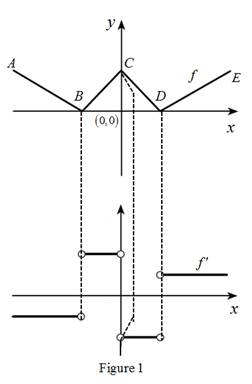# The graph of f ′ below the graph of f.### Single Variable Calculus: Concepts...

4th Edition
James Stewart
Publisher: Cengage Learning
ISBN: 9781337687805### Single Variable Calculus: Concepts...

4th Edition
James Stewart
Publisher: Cengage Learning
ISBN: 9781337687805

#### Solutions

Chapter 2.7, Problem 9E
To determine

## To sketch: The graph of f′ below the graph of f.

Expert Solution

### Explanation of Solution

From the graph, it is observed that the graph has a corner point at three points.

Let the three points be A, B and C. The derivative graph f is discontinuous at these points. That is, the derivative does not exist at these three points.

The graph from point A to B has negative slope which implies that the derivative graph must have negative value in this section. Since the function is linear in this part,

the derivative graph will be horizontal line.

The graph from point B to C has positive slope which implies that the derivative graph must have positive value in this section. Since the function is linear in this part,

the derivative graph will be horizontal line.

The graph from point C to D has negative slope which implies that the derivative graph must have a negative functional value. Since the function is linear in this part,

the derivative graph will be horizontal line.

The graph from point D to E has positive slope which implies that the derivative graph must have positive functional value. Since the function is linear in this part,

the derivative graph will be horizontal line.

Graph:

Use the above information and trace the graph of f(x) as shown below in Figure 1.Thus,f is the required graph.

### Have a homework question?

Subscribe to bartleby learn! Ask subject matter experts 30 homework questions each month. Plus, you’ll have access to millions of step-by-step textbook answers!